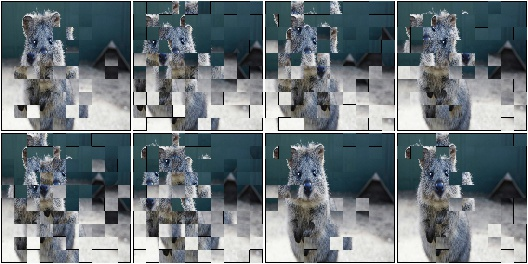# augmenters.geometric¶

## Affine¶

Augmenter to apply affine transformations to images.

API link: `Affine`

Example. Scale images to a value of 50 to 150% of their original size:

```import imgaug.augmenters as iaa
aug = iaa.Affine(scale=(0.5, 1.5))
```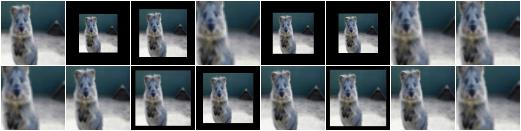Example. Scale images to a value of 50 to 150% of their original size, but do this independently per axis (i.e. sample two values per image):

```aug = iaa.Affine(scale={"x": (0.5, 1.5), "y": (0.5, 1.5)})
```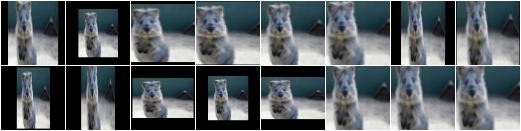Example. Translate images by -20 to +20% on x- and y-axis independently:

```aug = iaa.Affine(translate_percent={"x": (-0.2, 0.2), "y": (-0.2, 0.2)})
```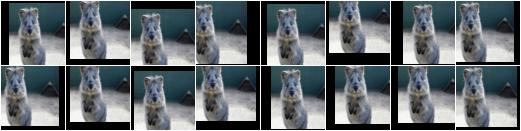Example. Translate images by -20 to 20 pixels on x- and y-axis independently:

```aug = iaa.Affine(translate_px={"x": (-20, 20), "y": (-20, 20)})
```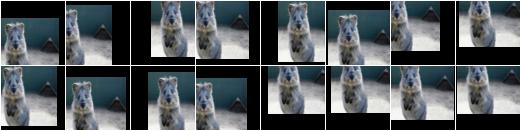Example. Rotate images by -45 to 45 degrees:

```aug = iaa.Affine(rotate=(-45, 45))
```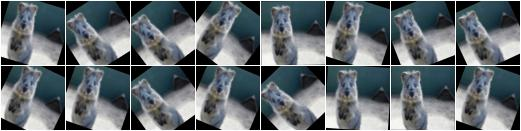Example. Shear images by -16 to 16 degrees:

```aug = iaa.Affine(shear=(-16, 16))
```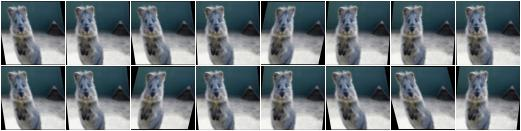Example. When applying affine transformations, new pixels are often generated, e.g. when translating to the left, pixels are generated on the right. Various modes exist to set how these pixels are ought to be filled. Below code shows an example that uses all modes, sampled randomly per image. If the mode is `constant` (fill all with one constant value), then a random brightness between 0 and 255 is used:

```aug = iaa.Affine(translate_percent={"x": -0.20}, mode=ia.ALL, cval=(0, 255))
```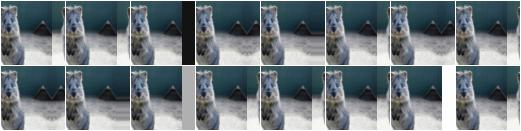## ScaleX¶

Apply affine scaling on the x-axis to input data.

This is a wrapper around `imgaug.augmenters.geometric.Affine`.

API link: `ScaleX`

Example. Create an augmenter that scales images along the width to sizes between `50%` and `150%`. This does not change the image shape (i.e. height and width), only the pixels within the image are remapped and potentially new ones are filled in.

```import imgaug.augmenters as iaa
aug = iaa.ScaleX((0.5, 1.5))
```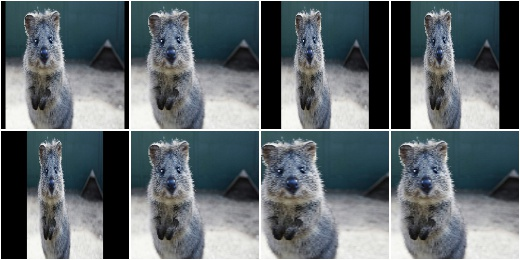## ScaleY¶

Apply affine scaling on the y-axis to input data.

This is a wrapper around `imgaug.augmenters.geometric.Affine`.

API link: `ScaleY`

Example. Create an augmenter that scales images along the height to sizes between `50%` and `150%`. This does not change the image shape (i.e. height and width), only the pixels within the image are remapped and potentially new ones are filled in.

```import imgaug.augmenters as iaa
aug = iaa.ScaleY((0.5, 1.5))
```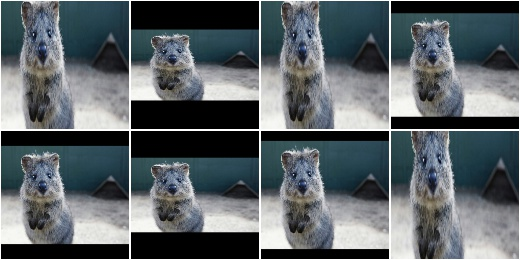## TranslateX¶

Apply affine translation on the x-axis to input data.

This is a wrapper around `imgaug.augmenters.geometric.Affine`.

API link: `TranslateX`

Example. Create an augmenter that translates images along the x-axis by `-20` to `20` pixels:

```import imgaug.augmenters as iaa
aug = iaa.TranslateX(px=(-20, 20))
```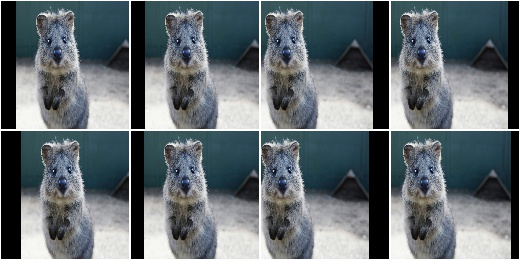Example. Create an augmenter that translates images along the x-axis by `-10%` to `10%` (relative to the x-axis size):

```aug = iaa.TranslateX(percent=(-0.1, 0.1))
```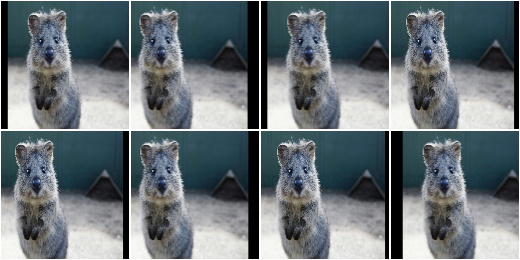## TranslateY¶

Apply affine translation on the y-axis to input data.

This is a wrapper around `imgaug.augmenters.geometric.Affine`.

API link: `TranslateY`

Example. Create an augmenter that translates images along the y-axis by `-20` to `20` pixels:

```import imgaug.augmenters as iaa
aug = iaa.TranslateY(px=(-20, 20))
```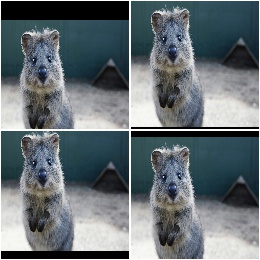Example. Create an augmenter that translates images along the y-axis by `-10%` to `10%` (relative to the y-axis size):

```aug = iaa.TranslateY(percent=(-0.1, 0.1))
```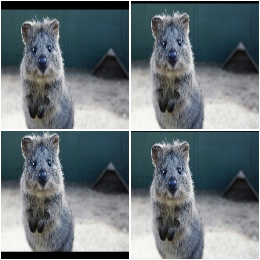## Rotate¶

Apply affine rotation on the y-axis to input data.

This is a wrapper around `imgaug.augmenters.geometric.Affine`. It is the same as `Affine(rotate=<value>)`.

API link: `Rotate`

Example. Create an augmenter that rotates images by a random value between `-45` and `45` degress:

```import imgaug.augmenters as iaa
aug = iaa.Rotate((-45, 45))
```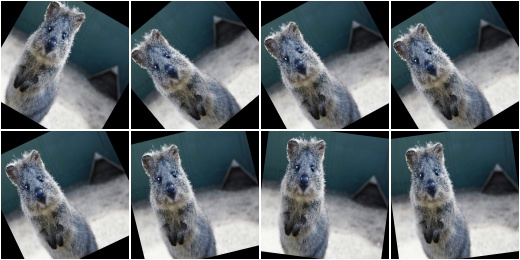## ShearX¶

Apply affine shear on the x-axis to input data.

This is a wrapper around `Affine`.

API link: `ShearX`

Example. Create an augmenter that shears images along the x-axis by random amounts between `-20` and `20` degrees:

```import imgaug.augmenters as iaa
aug = iaa.ShearX((-20, 20))
```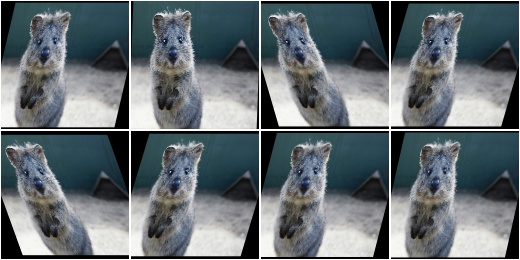## ShearY¶

Apply affine shear on the y-axis to input data.

This is a wrapper around `Affine`.

API link: `ShearY`

Example. Create an augmenter that shears images along the y-axis by random amounts between `-20` and `20` degrees:

```import imgaug.augmenters as iaa
aug = iaa.ShearY((-20, 20))
```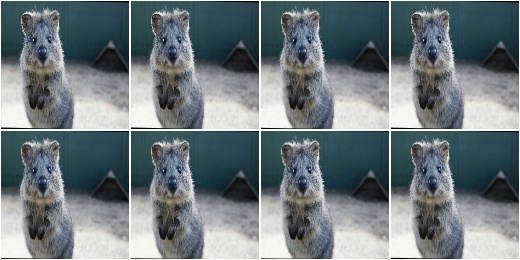## PiecewiseAffine¶

Apply affine transformations that differ between local neighbourhoods.

This augmenter places a regular grid of points on an image and randomly moves the neighbourhood of these point around via affine transformations. This leads to local distortions.

This is mostly a wrapper around scikit-image’s `PiecewiseAffine`. See also `Affine` for a similar technique.

Note

This augmenter is very slow. See Performance. Try to use `ElasticTransformation` instead, which is at least 10x faster.

Note

For coordinate-based inputs (keypoints, bounding boxes, polygons, …), this augmenter still has to perform an image-based augmentation, which will make it significantly slower for such inputs than other augmenters. See Performance.

API link: `PiecewiseAffine`

Example. Distort images locally by moving points around, each with a distance v (percent relative to image size), where v is sampled per point from `N(0, z)` `z` is sampled per image from the range 0.01 to 0.05:

```import imgaug.augmenters as iaa
aug = iaa.PiecewiseAffine(scale=(0.01, 0.05))
```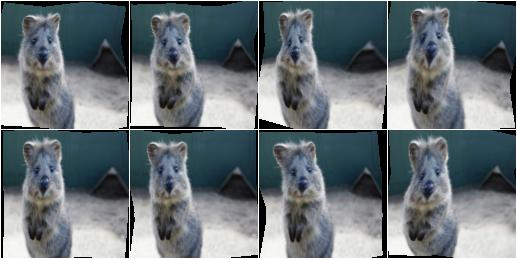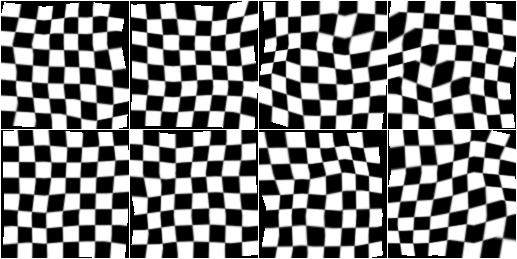Example. Effect of increasing `scale` from `0.01` to `0.3` in eight steps: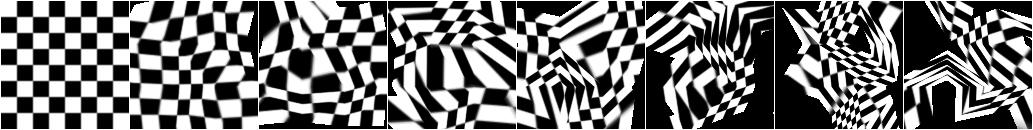Example. PiecewiseAffine works by placing a regular grid of points on the image and moving them around. By default this grid consists of 4x4 points. The below image shows the effect of increasing that value from 2x2 to 16x16 in 8 steps: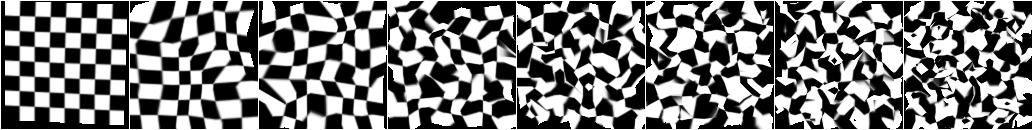## PerspectiveTransform¶

Apply random four point perspective transformations to images.

Each of the four points is placed on the image using a random distance from its respective corner. The distance is sampled from a normal distribution. As a result, most transformations don’t change the image very much, while some “focus” on polygons far inside the image.

The results of this augmenter have some similarity with `Crop`.

API link: `PerspectiveTransform`

Example. Apply perspective transformations using a random scale between `0.01` and `0.15` per image, where the scale is roughly a measure of how far the perspective transformation’s corner points may be distanced from the image’s corner points:

```import imgaug.augmenters as iaa
aug = iaa.PerspectiveTransform(scale=(0.01, 0.15))
```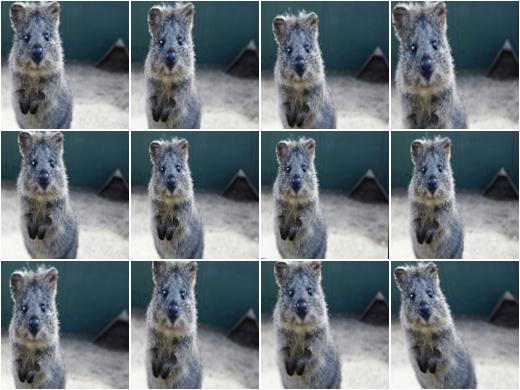Example. Same as in the previous example, but images are not resized back to the input image size after augmentation. This will lead to smaller output images.

```aug = iaa.PerspectiveTransform(scale=(0.01, 0.15), keep_size=False)
```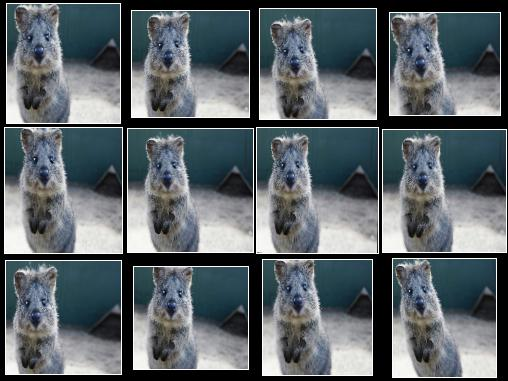`PerspectiveTransform` with `keep_size` set to `False`. Note that the individual images are here padded after augmentation in order to align them in a grid (i.e. purely for visualization purposes).

## ElasticTransformation¶

Transform images by moving pixels locally around using displacement fields.

The augmenter has the parameters `alpha` and `sigma`. `alpha` controls the strength of the displacement: higher values mean that pixels are moved further. `sigma` controls the smoothness of the displacement: higher values lead to smoother patterns – as if the image was below water – while low values will cause indivdual pixels to be moved very differently from their neighbours, leading to noisy and pixelated images.

A relation of 10:1 seems to be good for `alpha` and `sigma`, e.g. `alpha=10` and `sigma=1` or `alpha=50`, `sigma=5`. For `128x128` a setting of `alpha=(0, 70.0)`, `sigma=(4.0, 6.0)` may be a good choice and will lead to a water-like effect.

For a detailed explanation, see

```Simard, Steinkraus and Platt
Best Practices for Convolutional Neural Networks applied to Visual
Document Analysis
in Proc. of the International Conference on Document Analysis and
Recognition, 2003
```

Note

For coordinate-based inputs (keypoints, bounding boxes, polygons, …), this augmenter still has to perform an image-based augmentation, which will make it significantly slower for such inputs than other augmenters. See Performance.

API link: `ElasticTransformation`

Example. Distort images locally by moving individual pixels around following a distortions field with strength 0.25. The strength of the movement is sampled per pixel from the range 0 to 5.0:

```import imgaug.augmenters as iaa
aug = iaa.ElasticTransformation(alpha=(0, 5.0), sigma=0.25)
```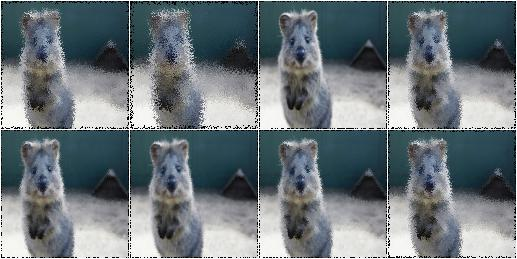Example. Effect of keeping sigma fixed at `0.25` and increasing alpha from `0` to `5.0` in eight steps: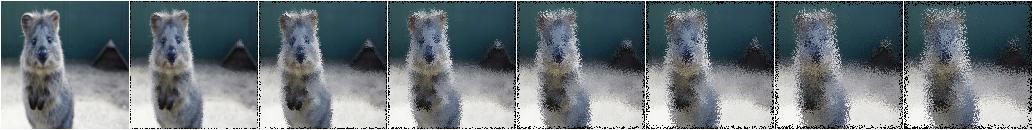Example. Effect of keeping alpha fixed at `2.5` and increasing sigma from `0.01` to `1.0` in eight steps: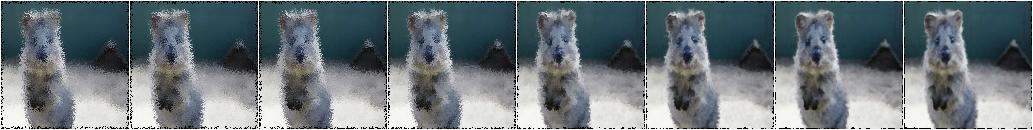## Rot90¶

Rotate images clockwise by multiples of 90 degrees.

This could also be achieved using `Affine`, but `Rot90` is significantly more efficient.

API link: `Rot90`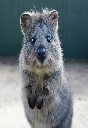The below examples use this input image, which slightly deviates from the examples for other augmenters (i.e. it is not square).

Example. Rotate all images by 90 degrees. Resize these images afterwards to keep the size that they had before augmentation. This may cause the images to look distorted.

```import imgaug.augmenters as iaa
aug = iaa.Rot90(1)
```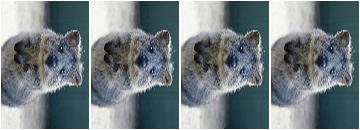Example. Rotate all images by 90 or 270 degrees. Resize these images afterwards to keep the size that they had before augmentation. This may cause the images to look distorted.

```aug = iaa.Rot90([1, 3])
```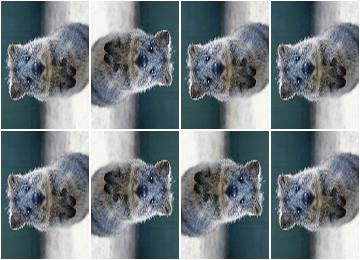Example. Rotate all images by 90, 180 or 270 degrees. Resize these images afterwards to keep the size that they had before augmentation. This may cause the images to look distorted.

```aug = iaa.Rot90((1, 3))
```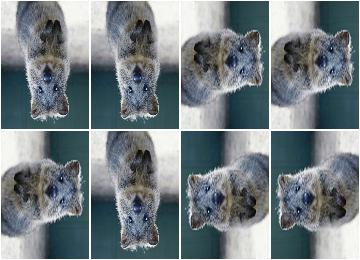Example. Rotate all images by 90, 180 or 270 degrees. Does not resize to the original image size afterwards, i.e. each image’s size may change.

```aug = iaa.Rot90((1, 3), keep_size=False)
```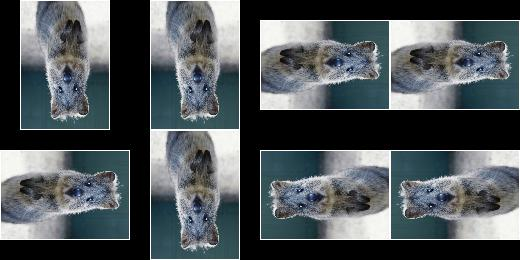`Rot90` with `keep_size` set to `False`. Note that the individual images are here padded after augmentation in order to align them in a grid (i.e. purely for visualization purposes).

## WithPolarWarping¶

Augmenter that applies other augmenters in a polar-transformed space.

This augmenter first transforms an image into a polar representation, then applies its child augmenter, then transforms back to cartesian space. The polar representation is still in the image’s input dtype (i.e. `uint8` stays `uint8`) and can be visualized. It can be thought of as an “unrolled” version of the image, where previously circular lines appear straight. Hence, applying child augmenters in that space can lead to circular effects. E.g. replacing rectangular pixel areas in the polar representation with black pixels will lead to curved black areas in the cartesian result.

This augmenter can create new pixels in the image. It will fill these with black pixels. For segmentation maps it will fill with class id `0`. For heatmaps it will fill with `0.0`.

This augmenter is limited to arrays with a height and/or width of `32767` or less.

Warning

When augmenting coordinates in polar representation, it is possible that these are shifted outside of the polar image, but are inside the image plane after transforming back to cartesian representation, usually on newly created pixels (i.e. black backgrounds). These coordinates are currently not removed. It is recommended to not use very strong child transformations when also augmenting coordinate-based augmentables.

Warning

For bounding boxes, this augmenter suffers from the same problem as affine rotations applied to bounding boxes, i.e. the resulting bounding boxes can have unintuitive (seemingly wrong) appearance. This is due to coordinates being “rotated” that are inside the bounding box, but do not fall on the object and actually are background. It is recommended to use this augmenter with caution when augmenting bounding boxes.

Warning

For polygons, this augmenter should not be combined with augmenters that perform automatic polygon recovery for invalid polygons, as the polygons will frequently appear broken in polar representation and their “fixed” version will be very broken in cartesian representation. Augmenters that perform such polygon recovery are currently `PerspectiveTransform`, `PiecewiseAffine` and `ElasticTransformation`.

API link: `WithPolarWarping`

Example. Apply cropping and padding in polar representation, then warp back to cartesian representation:

```import imgaug.augmenters as iaa
```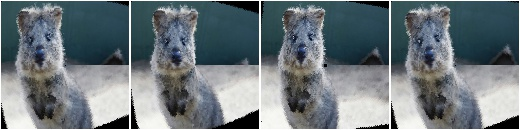Example. Apply affine translations in polar representation:

```aug = iaa.WithPolarWarping(
iaa.Affine(
translate_percent={"x": (-0.1, 0.1), "y": (-0.1, 0.1)}
)
)
```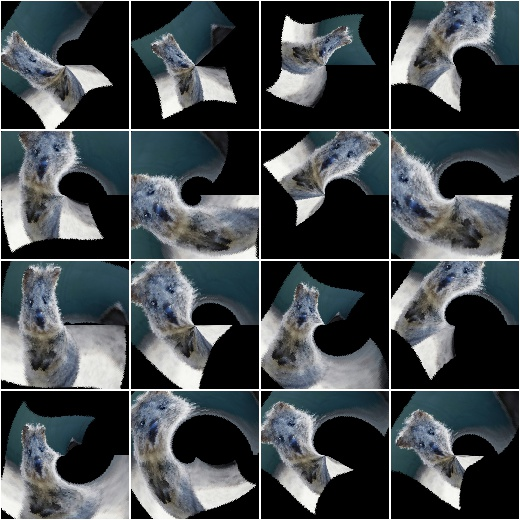Example. Apply average pooling in polar representation. This leads to circular bins:

```aug = iaa.WithPolarWarping(iaa.AveragePooling((2, 8)))
```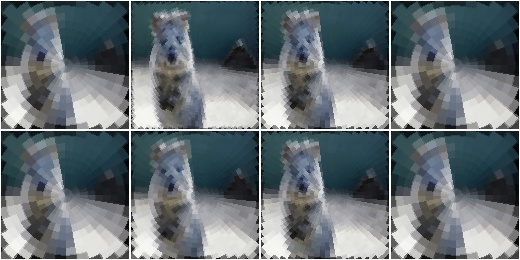## Jigsaw¶

Move cells within images similar to jigsaw patterns.

Note

This augmenter will by default pad images until their height is a multiple of nb_rows. Analogous for nb_cols.

Note

This augmenter will resize heatmaps and segmentation maps to the image size, then apply similar padding as for the corresponding images and resize back to the original map size. That also means that images may change in shape (due to padding), but heatmaps/segmaps will not change. For heatmaps/segmaps, this deviates from pad augmenters that will change images and heatmaps/segmaps in corresponding ways and then keep the heatmaps/segmaps at the new size.

Warning

This augmenter currently only supports augmentation of images, heatmaps, segmentation maps and keypoints. Other augmentables, i.e. bounding boxes, polygons and line strings, will result in errors.

API link: `Jigsaw`

Example. Create a jigsaw augmenter that splits images into `10x10` cells and shifts them around by `0` to `2` steps (default setting):

```import imgaug.augmenters as iaa
aug = iaa.Jigsaw(nb_rows=10, nb_cols=10)
```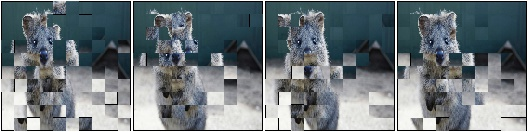Example. Create a jigsaw augmenter that splits each image into `1` to `4` cells along each axis:

```aug = iaa.Jigsaw(nb_rows=(1, 4), nb_cols=(1, 4))
```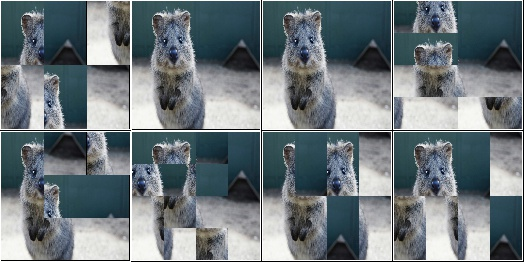Example. Create a jigsaw augmenter that moves the cells in each image by a random amount between `1` and `5` times (decided per image). Some images will be barely changed, some will be fairly distorted.

```aug = iaa.Jigsaw(nb_rows=10, nb_cols=10, max_steps=(1, 5))
```